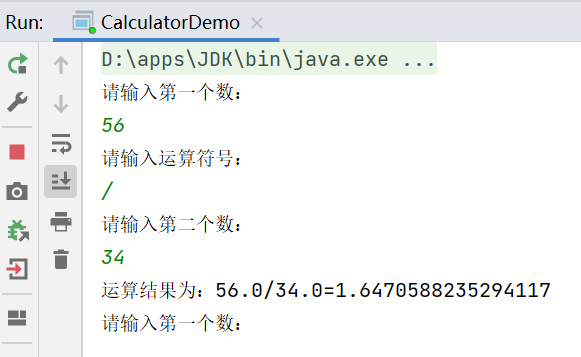# Java方法01

## 1.什么是方法?

• Java是语句的集合，它们在一起执行一个功能

• 方法是解决一类问题的步骤的有序集合

• 方法包含于类或者对象中

• 方法在程序中被创建，在其他地方被引用

• 设计方法的原则：方法的本意是功能块，就是实现某个功能的语句块的集合。我们设计方法的时候，最好保持方法的原子性，就是一个方法只完成一个功能，这样利于我们后期拓展。

• 方法的命名规则：首字母小写和驼峰原则：除了第一个单词外，之后的单词首字母大写

## 2.方法的定义和调用

### 2.1方法的定义

• Java的方法类似其他语言的函数，是一段用来完成特定功能的代码片段。一般情况下，定义一个方法包括以下语法：

• 方法包含一个方法头和一个方法体。下面是一个方法的所有部分：

• 修饰符:修饰符，这是可选的，告诉编译器如何调用该方法。定义了该方法的访问类型。
• 返回值类型：方法可能会返回值，returnValueType是方法返回值的数据类型。有些方法执行所需操作，但没有返回值， 这种情况下returnValueType是关键字void
• 方法名：是方法的实际名称，方法名和参数表共同构成方法签名。
• 参数类型：参数像是一个占位符，当方法被调用时，传递值给参数。这个值被称为实参或者变量。参数列表是指方法的参数类型、顺序和参数的个数。参数是可选的，方法也可以不包含任何参数。
• 形式参数：在方法被调用时用于接收外界输入的数据。
• 实参：调用方法时实际传给方法的数据。
• 方法体：方法体包含具体的语句，定义该方法的功能。

``````   修饰符 返回值类型 方法名(参数类型 参数名){
...
方法体
...
return 返回值;
}
``````

`````````java
package li.bolog.method;

public class Demo02 {
public static void main(String[] args) {
int max=max(20,20);
System.out.println(max);

}

//比大小
public static int max(int num1,int num2){
int result=0;
if(num1==num2){
return 0;   //return 除了可以返回值以外还可以终止方法
}
if (num1>num2) {
result=num1;
} else{
result=num2;
}
return  result;
}
}
```
``````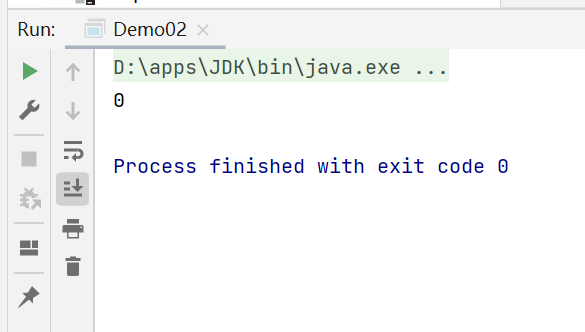### 2.3方法调用

• 调用方法：对象名.方法名(实参列表)

• Java支持两种调用方法的方式，根据方法是否返回值来选择

• 当方法返回一个值的时候，方法调用通常被当成一个值。例如：

`int largr = max(30,40);`

• 如果方法返回值是void，那么方法调用一定是一条语句。

`System.out.println("Hello");`

• 拓展了解：值传递 和 引用传递（PS：Java都是值传递）

## 3.方法的重载

• 重载就是在一个类中，有相同的函数名称，但形参不同的函数

• 方法的重载规则：

• 方法名称必须相同
• 参数列表可以不同（个数不同、类型不同、参数排列顺序不同等）
• 方法的返回类型可以相同也可以不相同
• 仅仅返回类型不同不足以成为方法的重载
• 实现理论：方法名称相同时，编译器会根据调用方法的参数个数、参数类型等去逐个匹配，以选择对应的方法，如果匹配失败则编译器报错。

重写：重写是垂直关系，是子类和父类之间的关系

重载：重载是水平关系，是同一个类中方法之间的关系

``````package li.bolog.method;

public class Demo01 {

public static void main(String[] args) {
System.out.println(sum);
System.out.println(sum2);

}

//加法
public static int add(int a,int b){
return a+b;
}

public static int add(int a,int b,int c){
return a+b+c;
}

}
``````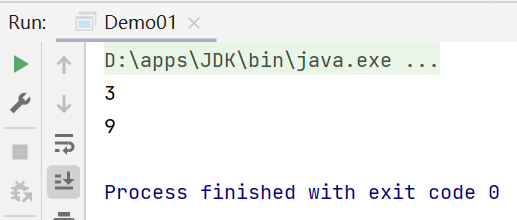## 4.命令行传参

``````package li.bolog.method;

public class Demo03 {
public static void main(String[] args) {
//args.length 数组长度
for (int i = 0; i < args.length; i++) {
System.out.println("args"+"["+i+"]"+args[i]);
}
}
}

``````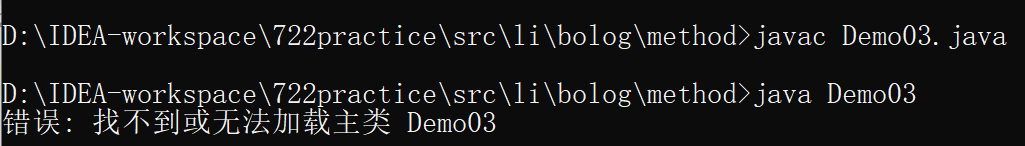``````D:\IDEA-workspace\722practice\src\li\bolog\method> cd ../
D:\IDEA-workspace\722practice\src\li\bolog> cd ../
D:\IDEA-workspace\722practice\src\li> cd ../
D:\IDEA-workspace\722practice\src> java li.bolog.method.Demo03
D:\IDEA-workspace\722practice\src> java li.bolog.method.Demo03 This is me
argsThis
argsis
argsme
``````

## 5.可变参数（不定项参数）

• 从JDK1.5开始，Java支持传递同类型的可变参数给一个方法
• 在方法声明中，在指定参数类型后面加一个省略号（…）
• 一个方法中只能指定一个可变参数，它必须是方法的最后一个参数。任何普通的参数必须在它之前声明

``````package li.bolog.method;

public class Demo04 {
public static void main(String[] args) {
printMax(2,9,55,7,8,56.7,-89);
printMax(new double[]{1,45,7,-9,77});
}

public static void printMax(double...numbers){
if(numbers.length==0){
System.out.println("No argument passed");
return;
}
double result = numbers;
//排序
for (int i = 1; i < numbers.length; i++) {
if(numbers[i]>result){
result = numbers[i];
}
}
System.out.println("The max value is"+result);
}
}
``````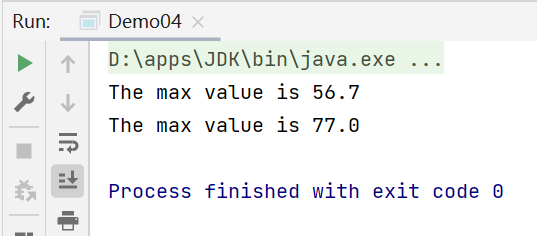## 6.递归

• A方法调用B方法，我们很容易理解。

递归就是：A方法调用A方法，就是自己调用自己。

• 利用递归可以用简单程序的程序来解决一些复杂的问题。它通常把一个大型的复杂的问题层层转化为一个与原问题相似的规模较小的问题来求解，递归策略只需少量的程序就可以描述出解题过程所需要的多次重复计算，大大减少了程序的代码量。递归的能力在于用有限的语句定义对象的无限集合。

• 递归结构包括两个部分：

• 递归头（递归边界）：什么时候不调用自身方法。如果没有头，就会陷入死循环。
• 递归体：什么时候需要调用自身方法
``````package li.bolog.method;

public class Demo05 {
public static void main(String[] args) {

System.out.println(f(5));
}
//递归来解决阶乘
//5!
public static int f(int n){
if(n==1){
return 1;
}else{
return n*f(n-1);
}
}
}
``````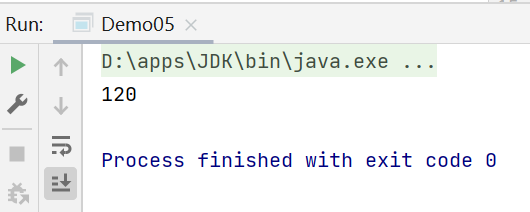## 7.巩固练习

• 思路推荐
• 写四个方法：加减乘除
• 利用循环+switch进行用户交互
• 传递需要操作的两个数
• 输出结果
``````package li.bolog.method;

import java.util.Scanner;

public class CalculatorDemo {
public static void main(String[] args) {
/*
写一个计算器，要求实现加减乘除功能，并且能够循环接收新的数据，通过用户交互实现。
- 思路推荐
- 写四个方法：加减乘除
- 利用循环+switch进行用户交互
- 传递需要操作的两个数
- 输出结果
* */

Scanner scanner = new Scanner(System.in);

double a = 0 ;
double b = 0 ;
String c =" " ;

for (int i = 0; i < 999; i++) {

System.out.println("请输入第一个数：");
a   =  scanner.nextDouble();

System.out.println("请输入运算符号：");
c   =  scanner.next();

System.out.println("请输入第二个数：");
b   =  scanner.nextDouble();

switch(c){
case "+" :

case "-" :
System.out.println("运算结果为："+a+"-"+b+"="+subtract(a,b));break;

case "*" :
System.out.println("运算结果为："+a+"*"+b+"="+multiply(a,b));break;

case "/" :
System.out.println("运算结果为："+a+"/"+b+"="+divide(a,b));break;

default:
System.out.println("请输入正确的运算符号！");
}
}

scanner.close();
}

//加
public static double add(double a,double b){return a+b;}

//减
public static double subtract(double a,double b){return a-b;}

//乘
public static double multiply(double a,double b){return a*b;}

//除
public static double divide(double a,double b){
if(a*b==0) {System.out.println("被除数或者除数不能为0！");return 0;}
else return a/b;
}
}
``````